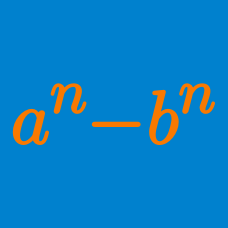Algebra

# Algebraic Manipulation

Consider the number

$N = \overline{123456789101112\ldots 9899100},$

which is obtained from writing all the integers from 1 to 100 and removing the spaces that are in between. What are the first 3 digits of $N^2$?

Evaluate

$\lfloor \left( \sqrt{ 23 } + \sqrt{17 } \right)^4 \rfloor .$

If the quartic $x^4 + 9x^3 + 11 x^2 + 36 x + A$ has roots $k, l, m$ and $n$ such that $kl = mn$, determine $A$.

Integers $N$ and $M$ satisfy $0 \leq M \leq 21!$ and

$\sum_{i=1}^{21} (i^2 + i + 1) i ! = N \times 21 ! - M.$

What is $N + M$?

If $x = \sqrt{ 11 + \sqrt{ 7 } }$ and $y = \sqrt{ 11 - \sqrt{ 7 } }$, evaluate

$x^6 + y^6.$

×